×#### Thank you for registering.

One of our academic counsellors will contact you within 1 working day.

Click to Chat

1800-1023-196

+91-120-4616500

CART 0

• 0

MY CART (5)

Use Coupon: CART20 and get 20% off on all online Study Material

ITEM
DETAILS
MRP
DISCOUNT
FINAL PRICE
Total Price: Rs.

There are no items in this cart.
Continue Shopping• Complete JEE Main/Advanced Course and Test Series
• OFFERED PRICE: Rs. 15,900
• View Details

```Chapter 14: Quadrilaterals Exercise – 14.3

Question: 1

In a parallelogram ABCD, determine the sum of angles ∠C and ∠D.

Solution:∠C and ∠D are consecutive interior angles on the same side of the transversal CD.

∴ ∠C + ∠D = 180°

Question: 2

In a parallelogram ABCD, if ∠B = 135°, determine the measures of its other angles.

Solution:

Given ∠B = 135°

ABCD is a parallelogram

∴ ∠A = ∠C, ∠B = ∠D and ∠A + ∠B = 180°

⇒ ∠A + 135° = 180°

⇒ ∠A = 45°

⇒ ∠A = ∠C = 45° and ∠B = ∠C = 135°

Question: 3

ABCD is a square. AC and BD intersect at O. State the measure of ∠AOB.

Solution: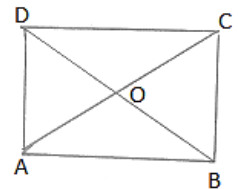Since, diagonals of a square bisect each other at right angle.

∴ ∠AOB = 90°

Question: 4

ABCD is a rectangle with ∠ABD = 40°. Determine ∠DBC

Solution: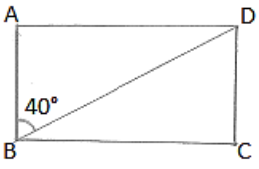We have,

∠ABC = 90°

⇒ ∠ABD + ∠DBC = 90° [∵ ∠ABD = 40°]

⇒ 400 + ∠DBC = 90°

∴ ∠DBC = 50°

Question: 5

The sides AB and CD of a parallelogram ABCD are bisected at E and F. Prove that EBFD is a parallelogram.

Solution: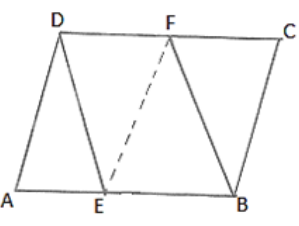Since ABCD is a parallelogram

∴ AB ∥ DC and AB = DC

⇒ EB ∥ DF and (1/2) AB = (1/2) DC

⇒ EB ∥ DF and EB = DF

EBFD is a parallelogram.

Question: 6

P and Q are the points of trisection of the diagonal BD of a parallelogram ABCD. Prove that CQ is parallel to AP. Prove also that AC bisects PQ.

Solution:

We know that,

Diagonals of a parallelogram bisect each other.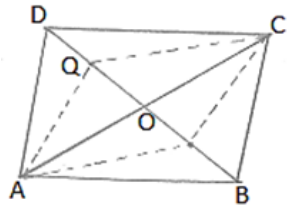Therefore, OA = OC and OB = OD

Since P and Q are point of intersection of BD.

Therefore, BP = PQ = QD

Now, OB = OD are BP = QD

⟹ OB - BP = OD - QD

⟹ OP = OQ

Thus in quadrilateral APCQ, we have

OA = OC and OP = OQ

Diagonals of Quadrilateral APCQ bisect each other.

Therefore APCQ is a parallelogram.

Hence AP ∥ CQ.

Question: 7

ABCD is a square. E, F, G and H are points on AB, BC, CD and DA respectively, such that AE = BF = CG = DH. Prove that EFGH is a square.

Solution:

We have,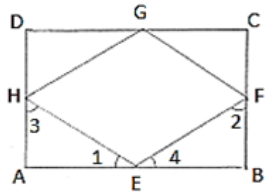AE = BF = CG = DH = x (say)

BE = CF = DG = AH = y (say)

In ΔAEH and ΔBEF, we have

AE = BF

∠A = ∠B

And AH = BE

So, by SAS congruency criterion, we have

ΔAEH ≃ ΔBFE

⇒ ∠1 = ∠2 and ∠3 = ∠4

But ∠1 + ∠3 = 90° and ∠2 + ∠A = 90°

⇒ ∠1 + ∠3 + ∠2 + ∠A = 90° + 90°

⇒ ∠1 + ∠4 + ∠1 + ∠4 = 180°

⇒ 2(∠1 + ∠4) = 180°

⇒ ∠1 + ∠4 = 90°

HEF = 90°

Similarly we have ∠F = ∠G = ∠H = 90°

Hence, EFGH is a Square.

Question: 8

ABCD is a rhombus, EAFB is a straight line such that EA = AB = BF. Prove that ED and FC when produced meet at right angles.

Solution:

We know that the diagonals of a rhombus are perpendicular bisector of each other.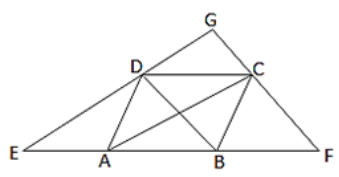∴ OA = OC, OB = OD, and ∠AOD = ∠COD = 90°

And ∠AOB = ∠COB = 90°

In ΔBDE, A and O are mid-points of BE and BD respectively.

OA ∥ DE

OC ∥ DG

In ΔCFA, B and O are mid-points of AF and AC respectively.

OB ∥ CF

OD ∥ GC

Thus, in quadrilateral DOGC, we have

OC ∥ DG and OD ∥ GC

⟹ DOCG is a parallelogram

∠DGC = ∠DOC

∠DGC = 90°

Question: 9

ABCD is a parallelogram, AD is produced to E so that DE = DC and EC produced meets AB produced in F. Prove that BF = BC.

Solution:

Draw a parallelogram ABCD with AC and BD intersecting at O.

Produce AD to E such that DE = DC

Join EC and produce it to meet AB produced at F.In ΔDCE,

∠DCE = ∠DEC ...  (i)  [In a triangle, equal sides have equal angles]

AB ∥ CD   [Opposite sides of the parallelogram are parallel]

∴ AE ∥ CD   [AB lies on AF]

AF∥CD and EF is the Transversal.

∠DCE = ∠BFC ... (ii)   [Pair of corresponding angles]

From (i) and (ii) we get

∠DEC = ∠BFC

In ΔAFE,

∠AFE = ∠AEF   [∠DEC = ∠BFC]

Therefore, AE = AF   [In a triangle, equal angles have equal sides opposite to them]

⟹ AD + DE = AB + BF

⟹ BC + AB = AB + BF   [Since, AD = BC, DE = CD and CD = AB, AB = DE]

⟹ BC = BF

Hence proved.
```### Course Features

• 728 Video Lectures
• Revision Notes
• Previous Year Papers
• Mind Map
• Study Planner
• NCERT Solutions
• Discussion Forum
• Test paper with Video Solution